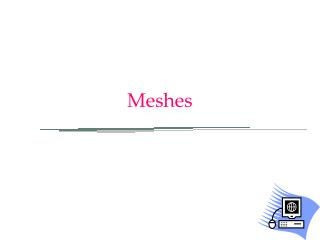Download PresentationMeshes

# Meshes - PowerPoint PPT PresentationDownload Presentation## Meshes

- - - - - - - - - - - - - - - - - - - - - - - - - - - E N D - - - - - - - - - - - - - - - - - - - - - - - - - - -
##### Presentation Transcript

1. Meshes

2. faces vertices Triangular Mesh (or mesh) • A mesh is a set of adjacent triangles edges

3. Topological properties of a mesh • Two Manifold or not • yes if each edge is shared by max 2 faces • two manifold = good • not two manifold = bad • most algorithms on meshes assume it is two-manifold NO YES

4. Topological properties of a mesh • Closed or open • When closed, each edge is shared by exactly two faces

5. Topological properties of a mesh • Coherent orientation • Is it possible to assign to each face an orientation so that the overall assignment is coherent? • Coherent orientation = coherent normals! A 1 1 3 D 2 3 2 C B Opposite direction, coherent edge

6. How can we define a mesh? • A mesh is a set of adjacent triangles • How can we define it? • Direct way: • An array of triangles • and for each triangle three vertices • and for each vertex three coordinate

7. How can we define a mesh? • Indexed mode • Ordered list of vertices • for each vertex its position • Ordered list of faces • for each face, 3 indices to vertices

8. What about the attributes? • Can be defined: • per vertex • Define an attribute for each vertex • per face • Define an attribute for each face • per wedge (vertex of face) • Define three attributes for each face • Most common attributes: • color • Texture coordinates • Normals

9. Example: PLY format • Digital format for meshes • Can be either binary, or ASCII (text) • binary: more compact and easy to machine-read • ascii: human-readable with a text-editor • In both cases, it starts with an ASCII header.

10. Example: PLY format cube.ply ply format ascii 1.0 comment a simple cube element vertex 8 property float x property float y property float z element face 12 property list uchar int vertex_indices end_header

11. # faces # edges # vertices first face: 4 vertices: with indices 3,2, 1 and 0 index 0 x,y,z 2nd vertex index 1 index 2 index 3 Example: OFF format LetterL.off OFF12 10 400 0 03 0 03 1 01 1 01 5 00 5 00 0 13 0 13 1 11 1 1 1 5 10 5 14 3 2 1 04 5 4 3 04 6 7 8 94 6 9 10 114 0 1 7 64 1 2 8 74 2 3 9 84 3 4 10 94 4 5 11 104 5 0 6 11

12. Mesh: common tasks • Given a mesh: compute the bounding box • Very useful in many applications, e.g. collisions • How to compute it? • We cycle on the vertices: compute the max and the min of all x, y and z

13. Mesh: common tasks • Given a mesh: compute the normals per vertex • How? • 1 Set all the vertex normals to 0 • 2 cycle on each face: • compute the normal • normalize it • add it to the vertex normal • 3 cycle on each vertex: • normalize the normal

14. More difficult tasks • Compute a Bounding sphere • Stripification • Parametrize • Automated simplification • Detail recovery • ...

15. More difficult tasks • Stripification • Organize the triangles in triangle strips • As long as possible

16. More difficult tasks • Parameterization • assign a pair of texture coordinates to each wedge • When there are seams (lines appearing in two separate images) • replicate the vertices • Save the texture coordinates for each wedge v

17. More difficult tasks • Automated simplification • Parameters: A maximal error or a given number of faces automatically mesh originale 500K triangles mesh semplificata 2K triangles

18. Automated simplification p e r f o r m a n c e q u a l i t y

19. Automated simplification A hierarchy of Levels of Detail LOD 2 LOD 3 LOD 4 LOD 1 To be used when viewer is far away To be used when viewer is nearby

20. Detail preservation (or texture for geometry) • Idea: • Synthesize a texture • To compensate for the detail lost during simplification

21. Ad-hoc created TEXTURE detail recovery rendering 2k triangles, with texture mapping 500k triangles Automated simplification 2k triangles

22. simplified but textured 2K triangles originale 500K triangles simplified 2K triangles